7. Poincaré-Lindstedt's method.

Poincaré-Lindstedt’s method is a method to avoid secular terms. Vi introducerar en “störd” tidwhereand putExample: We examine Duffing’s equationagain. By doing the change of variablesthe equation is transformed tosinceIf we insert the the expressions for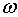and u in this we getandNow we compare powers of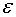: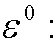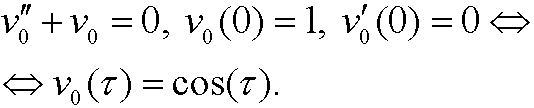By choosing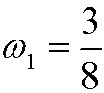we see that we can avoid the secular term. This leads towith the solutionAlltogether we have that a first order perturbation solution of Duffings equation iswhereRemark: Poincaré-Lindstedt’s method  works for some (not all) equations on the form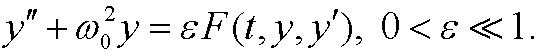Troubles arise when also the right hand side has the frequencyin some step. We will then not succeed with the particular solution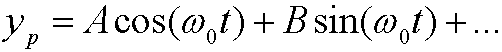but instead have to try with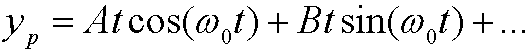which leads to secular terms. This trouble can be avoided in Poincaré-Lindstedt’s method by instead put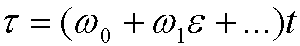and then solve the corresponding equations as usual.